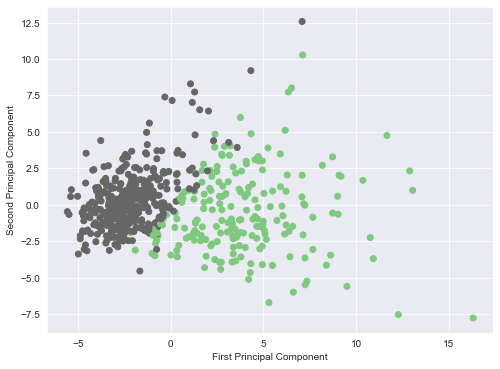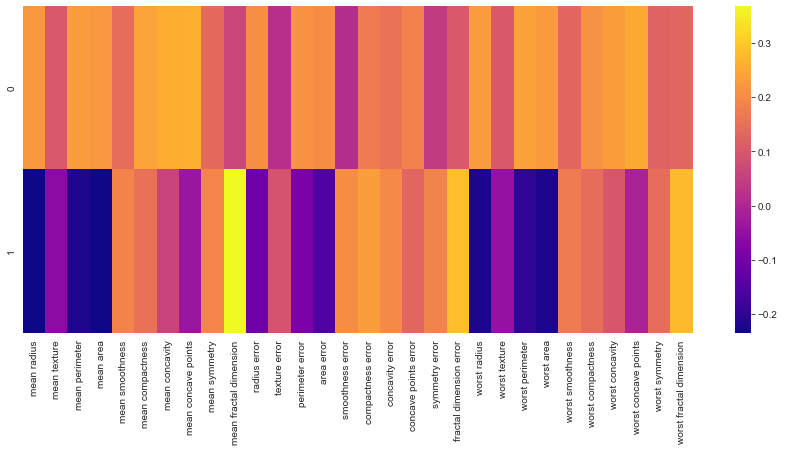The greatest variance is shown on an orthogonal line perpendicular to the axis. Likewise, the second greatest variation on the second axis, and so on.

This allows us to reduce the number of variables used in an analysis.

Taking this a step further – we can expand to higher level of dimensions – shown as “components”.

If we utilize a dataset with a large number of variables, this helps us reduce the amount of variation to a small number of components – but these can be tough to interpret. A much more detailed walk-through on the theory can be found here. I’m going to show how this analysis can be done utilizing Scikit learn in Python. The dataset were going to be utilizing can be loaded directly within sklearn as shown below.

## Principal Component Analysis – Simple Example

We’re going to be utilizing a cancer dataset that is found within sklearn. We’re going to try to find what components are most important (show the most variance) in this dataset.

In :
```#Imports
import pandas as pd
import numpy as np
import matplotlib.pyplot as plt
import seaborn as sns
%matplotlib inline
```

In :
```#Import Data
from sklearn.datasets import load_breast_cancer

```
In :
```#Some info about this dataset
print(cancer['DESCR'])
```
```.. _breast_cancer_dataset:

Breast cancer wisconsin (diagnostic) dataset
--------------------------------------------

**Data Set Characteristics:**

:Number of Instances: 569

:Number of Attributes: 30 numeric, predictive attributes and the class

:Attribute Information:
- radius (mean of distances from center to points on the perimeter)
- texture (standard deviation of gray-scale values)
- perimeter
- area
- smoothness (local variation in radius lengths)
- compactness (perimeter^2 / area - 1.0)
- concavity (severity of concave portions of the contour)
- concave points (number of concave portions of the contour)
- symmetry
- fractal dimension ("coastline approximation" - 1)

The mean, standard error, and "worst" or largest (mean of the three
worst/largest values) of these features were computed for each image,
resulting in 30 features.  For instance, field 0 is Mean Radius, field
10 is Radius SE, field 20 is Worst Radius.

- class:
- WDBC-Malignant
- WDBC-Benign

:Summary Statistics:

===================================== ====== ======
Min    Max
===================================== ====== ======
radius (mean):                        6.981  28.11
texture (mean):                       9.71   39.28
perimeter (mean):                     43.79  188.5
area (mean):                          143.5  2501.0
smoothness (mean):                    0.053  0.163
compactness (mean):                   0.019  0.345
concavity (mean):                     0.0    0.427
concave points (mean):                0.0    0.201
symmetry (mean):                      0.106  0.304
fractal dimension (mean):             0.05   0.097
radius (standard error):              0.112  2.873
texture (standard error):             0.36   4.885
perimeter (standard error):           0.757  21.98
area (standard error):                6.802  542.2
smoothness (standard error):          0.002  0.031
compactness (standard error):         0.002  0.135
concavity (standard error):           0.0    0.396
concave points (standard error):      0.0    0.053
symmetry (standard error):            0.008  0.079
fractal dimension (standard error):   0.001  0.03
radius (worst):                       7.93   36.04
texture (worst):                      12.02  49.54
perimeter (worst):                    50.41  251.2
area (worst):                         185.2  4254.0
smoothness (worst):                   0.071  0.223
compactness (worst):                  0.027  1.058
concavity (worst):                    0.0    1.252
concave points (worst):               0.0    0.291
symmetry (worst):                     0.156  0.664
fractal dimension (worst):            0.055  0.208
===================================== ====== ======

:Missing Attribute Values: None

:Class Distribution: 212 - Malignant, 357 - Benign

:Creator:  Dr. William H. Wolberg, W. Nick Street, Olvi L. Mangasarian

:Donor: Nick Street

:Date: November, 1995

This is a copy of UCI ML Breast Cancer Wisconsin (Diagnostic) datasets.
https://goo.gl/U2Uwz2

Features are computed from a digitized image of a fine needle
aspirate (FNA) of a breast mass.  They describe
characteristics of the cell nuclei present in the image.

Separating plane described above was obtained using
Multisurface Method-Tree (MSM-T) [K. P. Bennett, "Decision Tree
Construction Via Linear Programming." Proceedings of the 4th
Midwest Artificial Intelligence and Cognitive Science Society,
pp. 97-101, 1992], a classification method which uses linear
programming to construct a decision tree.  Relevant features
were selected using an exhaustive search in the space of 1-4
features and 1-3 separating planes.

The actual linear program used to obtain the separating plane
in the 3-dimensional space is that described in:
[K. P. Bennett and O. L. Mangasarian: "Robust Linear
Programming Discrimination of Two Linearly Inseparable Sets",
Optimization Methods and Software 1, 1992, 23-34].

This database is also available through the UW CS ftp server:

ftp ftp.cs.wisc.edu
cd math-prog/cpo-dataset/machine-learn/WDBC/

.. topic:: References

- W.N. Street, W.H. Wolberg and O.L. Mangasarian. Nuclear feature extraction
for breast tumor diagnosis. IS&T/SPIE 1993 International Symposium on
Electronic Imaging: Science and Technology, volume 1905, pages 861-870,
San Jose, CA, 1993.
- O.L. Mangasarian, W.N. Street and W.H. Wolberg. Breast cancer diagnosis and
prognosis via linear programming. Operations Research, 43(4), pages 570-577,
July-August 1995.
- W.H. Wolberg, W.N. Street, and O.L. Mangasarian. Machine learning techniques
to diagnose breast cancer from fine-needle aspirates. Cancer Letters 77 (1994)
163-171.
```
In :
```#Convert to dataframe
df = pd.DataFrame(cancer['data'],columns=cancer['feature_names'])
```
In :
```df.head()
```
Out:
mean radius mean texture mean perimeter mean area mean smoothness mean compactness mean concavity mean concave points mean symmetry mean fractal dimension worst radius worst texture worst perimeter worst area worst smoothness worst compactness worst concavity worst concave points worst symmetry worst fractal dimension
0 17.99 10.38 122.80 1001.0 0.11840 0.27760 0.3001 0.14710 0.2419 0.07871 25.38 17.33 184.60 2019.0 0.1622 0.6656 0.7119 0.2654 0.4601 0.11890
1 20.57 17.77 132.90 1326.0 0.08474 0.07864 0.0869 0.07017 0.1812 0.05667 24.99 23.41 158.80 1956.0 0.1238 0.1866 0.2416 0.1860 0.2750 0.08902
2 19.69 21.25 130.00 1203.0 0.10960 0.15990 0.1974 0.12790 0.2069 0.05999 23.57 25.53 152.50 1709.0 0.1444 0.4245 0.4504 0.2430 0.3613 0.08758
3 11.42 20.38 77.58 386.1 0.14250 0.28390 0.2414 0.10520 0.2597 0.09744 14.91 26.50 98.87 567.7 0.2098 0.8663 0.6869 0.2575 0.6638 0.17300
4 20.29 14.34 135.10 1297.0 0.10030 0.13280 0.1980 0.10430 0.1809 0.05883 22.54 16.67 152.20 1575.0 0.1374 0.2050 0.4000 0.1625 0.2364 0.07678

5 rows Ã— 30 columns

### Scale the data

We need to scale the data to put everything into a common scale before utilizing PCA

In :
```#Import scaler and scale
from sklearn.preprocessing import StandardScaler

#Instantiate object
scaler = StandardScaler()

#Fit df to scaler
scaler.fit(df)

#Scale data
scaled_data = scaler.transform(df)
```

### PCA – Find 2 principal components

In :
```from sklearn.decomposition import PCA
```
In :
```#Create object with 2 componenets
pca = PCA(n_components=2)
```
In :
```#Fit scaled data
pca.fit(scaled_data)
```
Out:
`PCA(n_components=2)`
In :
```#Transform
x_pca = pca.transform(scaled_data)
```
In :
```#Shows that we've reduced down to the 2 principal components
x_pca.shape
```
Out:
`(569, 2)`

### Plot the components

Utilizing the color of the ‘target’ column, which specifies if a tumor is benign or malignent. We can see a clear separation of the two components. We’re essentially ‘compressing’ the data and comparing the two components against all columns of data.

In :
```#Plot these
sns.set_style("darkgrid")

plt.figure(figsize=(8,6))
plt.scatter(x_pca[:,0],x_pca[:,1],c=cancer['target'],cmap='Accent')

plt.xlabel('First Principal Component')
plt.ylabel('Second Principal Component')
```
Out:
`Text(0, 0.5, 'Second Principal Component')`### Appendix: Other Considerations

How can we determine what dimensions of the data are more heavily weighted to one component vs. another?

In :
```#We have the component values here
pca.components_
```
Out:
```array([[ 0.21890244,  0.10372458,  0.22753729,  0.22099499,  0.14258969,
0.23928535,  0.25840048,  0.26085376,  0.13816696,  0.06436335,
0.20597878,  0.01742803,  0.21132592,  0.20286964,  0.01453145,
0.17039345,  0.15358979,  0.1834174 ,  0.04249842,  0.10256832,
0.22799663,  0.10446933,  0.23663968,  0.22487053,  0.12795256,
0.21009588,  0.22876753,  0.25088597,  0.12290456,  0.13178394],
[-0.23385713, -0.05970609, -0.21518136, -0.23107671,  0.18611302,
0.15189161,  0.06016536, -0.0347675 ,  0.19034877,  0.36657547,
-0.10555215,  0.08997968, -0.08945723, -0.15229263,  0.20443045,
0.2327159 ,  0.19720728,  0.13032156,  0.183848  ,  0.28009203,
-0.21986638, -0.0454673 , -0.19987843, -0.21935186,  0.17230435,
0.14359317,  0.09796411, -0.00825724,  0.14188335,  0.27533947]])```
In :
```#Set these to a new DataFrame
df_comp = pd.DataFrame(pca.components_,columns=cancer['feature_names'])
```
In :
```df_comp.head()
```
Out:
mean radius mean texture mean perimeter mean area mean smoothness mean compactness mean concavity mean concave points mean symmetry mean fractal dimension worst radius worst texture worst perimeter worst area worst smoothness worst compactness worst concavity worst concave points worst symmetry worst fractal dimension
0 0.218902 0.103725 0.227537 0.220995 0.142590 0.239285 0.258400 0.260854 0.138167 0.064363 0.227997 0.104469 0.236640 0.224871 0.127953 0.210096 0.228768 0.250886 0.122905 0.131784
1 -0.233857 -0.059706 -0.215181 -0.231077 0.186113 0.151892 0.060165 -0.034768 0.190349 0.366575 -0.219866 -0.045467 -0.199878 -0.219352 0.172304 0.143593 0.097964 -0.008257 0.141883 0.275339

2 rows Ã— 30 columns

#### Plotting a heatmap then shows you which dimension is more heavily weighted to which component. The higher the number, the more it correlates to a specific feature in the columns

In :
```plt.figure(figsize=(15,6))
sns.heatmap(df_comp,cmap='plasma')
```
Out:
`<matplotlib.axes._subplots.AxesSubplot at 0x21f314d5048>`### Appendix: What now?

Since we now have two components that are easily separable, we could feed this into a model instead of attempting to feed the original dataframe. And – this could be condusive for a Support Vector Machine (SVM) model given the two halves we plainly see. We could attempt that now since I already utilized this dataset in my other tutorial on SVM to see if it changes anything

https://www.mikekale.com/support-vector-machines-in-python-simple-example/

In :
```#Import splitting library
from sklearn.model_selection import train_test_split
```
In :
```#Set X,Y
X = x_pca
y = cancer['target']
```
In :
```#Choose the test size
#Test size = % of dataset allocated for testing (.3 = 30%)
#Random state = # of random splits
X_train, X_test, y_train, y_test = train_test_split(X, y, test_size=0.3, random_state=101)
```
In :
```#Import library
from sklearn.svm import SVC
```
In :
```#Create object
model = SVC()
```
In :
```#Fit
model.fit(X_train,y_train)
```
Out:
`SVC()`
In :
```#Predict
predictions = model.predict(X_test)
```
In :
```#See if the model worked, print reports (worked very well)
from sklearn.metrics import classification_report, confusion_matrix
```
In :
```print(confusion_matrix(y_test,predictions))
```
```[[ 56  10]
[  5 100]]
```
In :
```print(classification_report(y_test,predictions))
```
```              precision    recall  f1-score   support

0       0.92      0.85      0.88        66
1       0.91      0.95      0.93       105

accuracy                           0.91       171
macro avg       0.91      0.90      0.91       171
weighted avg       0.91      0.91      0.91       171

```

#### In this case – the model is great, but actually underperforms the original (non PCA version) a bit. Although nothing really improved in this case, it may certain help in other situations.

WordPress conversion from Principal Component Analysis – Simple Example.ipynb by nb2wp v0.3.1